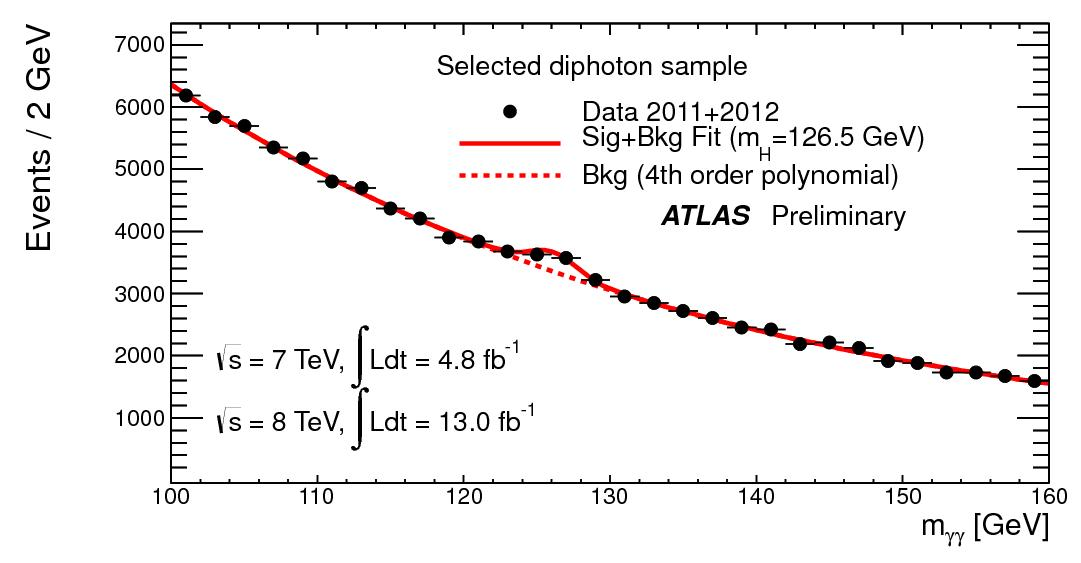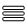Standard deviation

Taking a closer look at LHC

Far from the usual idea, searches in Particle Physics are not done in an “individual way”, butlooking for an “excess” above background, i.e., a series of events with the same properties (such as the mass). All this involves, therefore, an accurate statistical analysis of the data taken in the experiments.

Hence a “discovery” in this field involves knowing the amount of background to expect, and being sure that the excess, in case it is there, is not the result of a statistical fluctuation of this background. Indeed, we know that for a distribution with a given average, we will not always measure the value of the average: the measured value will fluctuate with respect to it. Let’s think in a shop which measures, along several months, the number of customers that come in each day. If the average is 100, in a random day, the number of customers going in does not have to be necessarily 100, although it is also true that the probability that this number is 1000 is very low. In summary, the statistical fluctuations can explain an excess (or decrease) with respect to the average, but only to some extent. If the measured value was 1000 we would be facing an “extraordinary” situation.

The scientific work requires then a quantification of these excesses. In particular, it is all about establishing the probability that an observed excess was a statistical fluctuation of the background. In this way, we speak about a  p-value or of a “number of σ”. The origin of the term “number of σ” comes from of the gaussian distribution that describes the behaviour of the background in many cases. The σ of a Gauss distribution corresponds to its width. In practice,the greater number of σ the minor will be the p-value or, what is the same, the lower will be the probability that an observed excess can be explained by a background fluctuation.

Notable values for an excess in particle physics are 3σ, or p-value = 0.0013; and 5σ, or p-value = 2.87 x 10-7When we have an excess of 3σ we talk about an evidence, and when we have an excess of 5σ, we are facing a discovery.

In the case of 5σ, the p-value indicates that the excess with respect to the background would be a statistical fluctuation only 1 time of each 3 million and half experiments[1/(2,87x10-7]. Indeed, we have a discovery

A new particle has been discovered at the LHC during 2012, and its properties are very similar to those of the Higgs boson. Let’s take as a process to study that in which this new particle (that we will call deliberately H) decays to two photons, i.e., the process in which H turns into two photons (H→γγ). But there are a lot of other processes that resemble this, and that constitute the background. On the other hand, for the pairs of two photons it is possible to reconstruct the mass of the supposed particle from which they proceed. The ATLAS collaboration, at CERN, did exactly this and represented the number of “candidates” in different regions of mass (see the following figure).Generally, it can be seen how the background follows a particular pattern, for which it is possible to fit a fourth order polynomial. However, in the region around 126.5 GeV/c2 an excess above the background is observed. The quantification of this excess gives 6.1σ, that corresponds to a p-value = 4.4 × 10−10. Or, what is the same, the probability that the observed excess was due to a statistical fluctuation of the background is of 4 each ten thousand millions. For every ten thousand million times that this experiment was done, only in 4 of them the background would have fluctuated so much! Therefore, since the excess surpasses widely the 5σ, there is no doubt that a new particle has been discovered. From now on, it becomes necessary to determine carefully which are his properties, to confirm that it is the Higgs boson.

 AUTHORS Xabier Cid Vidal, PhD in experimental Particle Physics for Santiago University (USC). Research Fellow in experimental Particle Physics at CERN from January 2013 to Decembre 2015. Currently, he is in USC Particle Physics Department ("Ramon y Cajal", Spanish Postdoctoral Senior Grants). Ramon Cid Manzano, secondary school Physics Teacher at IES de SAR (Santiago - Spain), and part-time Lecturer (Profesor Asociado) in Faculty of Education at the University of Santiago (Spain). He has a Degree in Physics and in Chemistry, and is PhD for Santiago University (USC). CERN CERN WEBSITE CERN Directory CERN Experimental Program Theoretical physics (TH) CERN Physics Department CERN Scientific Committees CERN Structure CERN and the Environment LHC LHC Detector CMS Detector ATLAS Detector ALICE Detector LHCb Detector TOTEM Detector LHCf Detector MoEDAL

IMPORTANT NOTICE

For the bibliography used when writing this Section please go to the References Section

© Xabier Cid Vidal & Ramon Cid - rcid@lhc-closer.es  | SANTIAGO (SPAIN) |